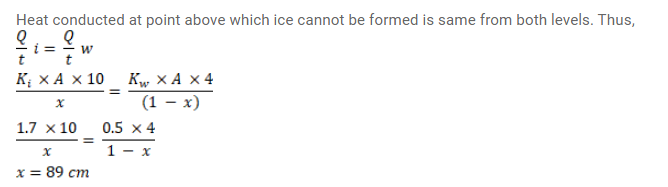# Consider the situation of the previous problem.`
Question:

Consider the situation of the previous problem. Assume that the temperature of the water at the bottom of the lake remains constant at $4^{\circ} \mathrm{C}$ as the ice forms on the surface (the heat required to maintain the temperature of the bottom layer may come from the bed of the lake). The depth of the lake is $1.0 \mathrm{~m}$. Show that the thickness of the ice formed attains a steady state maximum value. Find this value. The thermal conductivity of water $=0.50 \mathrm{~W} / \mathrm{m}-{ }^{\circ} \mathrm{C}$ Take other relevant data from the previous problem.

Solution: